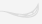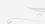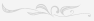## Меню

Главная
Финансы деньги и налоги
Издательское дело и полиграфия
Новейшая история политология
Топики по английскому языку
Языкознание филология
Химия
Экология и охрана природы
Естествознание
Искусство и культура
Культурология
Хозяйственное право
Иностранные языки и языкознание
История и исторические личности
Коммуникации связь цифровые приборы и радиоэлектроника
Товароведение
Транспорт
Экология охрана труда
Банковское и биржевое дело
Коммуникации и связь
Конституционное право
Этика

##### Научно-образовательный портал W-10.RUГлавная

# Mathematics as a science

## Mathematics as a science

Mathematics as a science, viewed as a whole, is a collection of branches.

The largest branch is that which builds on the ordinary whole numbers, fractions, and irrational numbers, or what, collectively, is called the real number system.

Arithmetic, algebra, the study of functions, the calculus, differential equations, and various other subjects which follow the calculus in logical order are all developments of the real number system.

This part of mathematics is termed the mathematics of number.

A second branch is geometry consisting of several geometries.

Mathematics contains many more divisions.

Each branch has the same logical structure: it begins with certain concepts, such as the whole numbers or integers in the mathematics of number, and such as point, line and triangle in geometry.

These concepts must verify explicitly stated axioms.

Some of the axioms of the mathematics of number are the associative, commutative, and distributive properties and the axioms about equalities.

Some of the axioms of geometry are that two points determine a line, all right angles are equal, etc.

From the concepts and axioms theorems are deduced.

Hence, from the standpoint of structure, the concepts, axioms and theorems are the essential components of any compartment of mathematics.

We must break down mathematics into separately taught subjects, but this compartmentalization taken as a necessity, must be compensated for as much as possible.

Students must see the interrelationships of the various areas and the importance of mathematics for other domains.

Knowledge is not additive but an organic whole and mathematics is an inseparable part of that whole.

The full significance of mathematics can be seen and taught only in terms of its intimate relationships to other fields of knowledge.

If mathematics is isolated from other provinces, it loses importance.

## Список литературы

Для подготовки данной работы были использованы материалы с сайта http://matfak.ru/## Поиск

Расширенный поиск